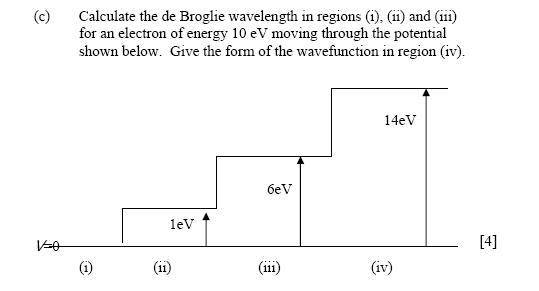# Potentials and de Broglie wavelength

## Homework Statement## Homework Equations

E=hc/λ?

and the Time Independent Schrodinger Equation.

## The Attempt at a Solution

Now, would I be right in thinking for the first section that the energy for the E=hc/λ bit is just the energy of the particle given in the question (10eV).

Then for the other sections is the relevant energy just 10eV minus the potential?

As the last section will give a negative KE of the particle does that mean that it doesn't have a de Broglie wavelength? Its just a negatively decaying exponential (i.e. tending to 0)?

Does that sound like I'm on the right lines?

Anyone have any hints about this one? The way that I described it above is the way that I attempted it, and after using the wrong values from my data sheet (first I used k(why I picked this I don't know - maybe I just can't read!) and then $$\hbar$$ before reading the sheet properly for the value of h!) I think I have values that give decent answers.

Dick
Homework Helper
It sounds fine to me. Decaying exponential in the last region, yes. Is there still a question?

Gokul43201
Staff Emeritus
Gold Member

## Homework Statement## Homework Equations

E=hc/λ?
Are we talking about photons or electrons? What is the general de Broglie relation for any particle?